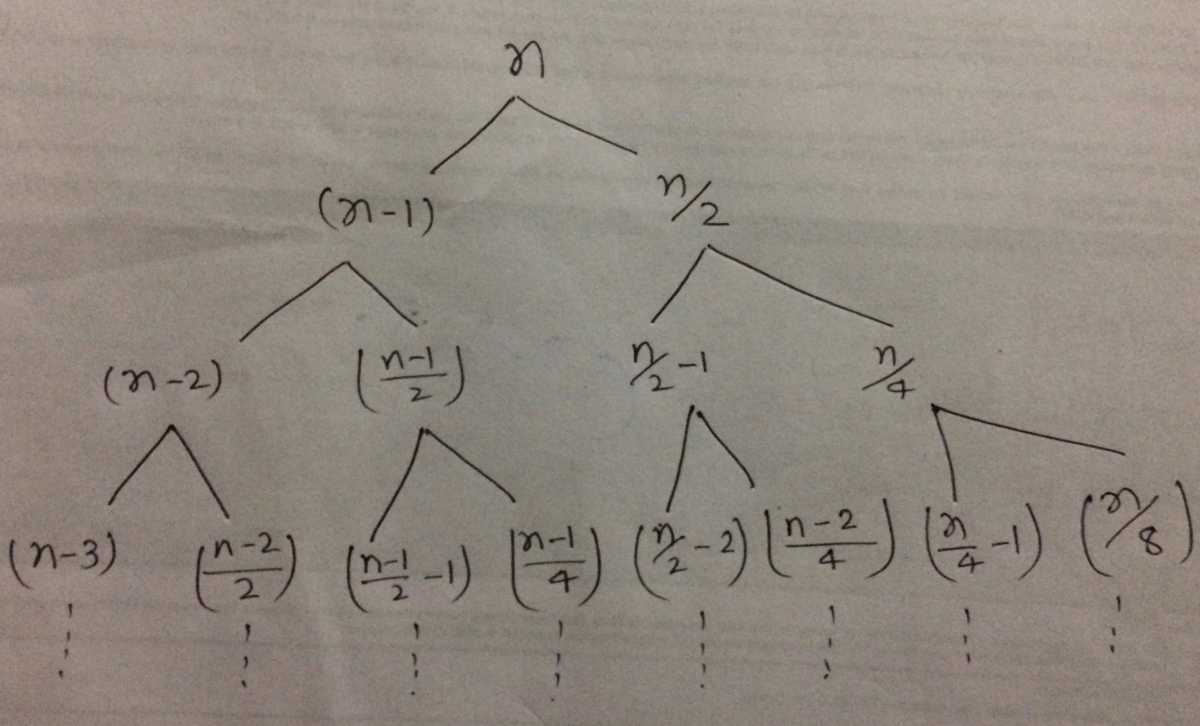##### Find the big O complexity for the given Reccurence relation

how can i calulate big O complexity for

T(n) =T(n-1) + T(n/2) +n

Please explain in detail

shivani
18 Aug 2017 11:21 am

T(n) =T(n-1) + T(n/2) +n can also be written as T(n) = f(n) + g(n)

f(n) = f(n-1) +n and g(n) = g(n/2) +n

after solving both , we get complexity of f(n) as O(n2) and complexity of g(n) as O(log n)
So , complexity of T(n) is worst case of O(n2) and O(log n) , thus time complexity is O(n2)

Arpit Dhuriya
19 Aug 2017 08:36 pm
just take the one which is asymptotically larger. here t(n/2) will be consumed before the t(n-1) so for taking that only we can conclude t(n) ~== t(n-1) + n
Parth Sharma
19 Aug 2017 08:56 pm

Thanks a lot arpit .

I know this but if u r doing this then it is omega not big o .

I need to know the answer and even i know the answer i wanted to know the approach.

Pritam Prasun
19 Aug 2017 09:32 pm

Let us apply the recursion-tree method for solving this recurrence problem:The cost of the root is n because it is the combined cost to divide and merge back the results from the conquered subprocesses. Now here you can see that the height of the subtrees will be largest for T(n-1). It is equivalent to the fact mentioned by @arpitdhuriya that T(n-1) is asymptotically larger.

Hence the complexity would be O(n2)
More precisely, Ω(n2)